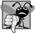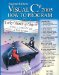# Variable-Length Argument Lists

Variable-length argument lists allow you to create methods that receive an arbitrary number of arguments. A one-dimensional array-type argument preceded by the keyword params in a method's parameter list indicates that the method receives a variable number of arguments with the type of the array's elements. This use of a params modifier can occur only in the last entry of the parameter list. While you can use method overloading and array passing to accomplish much of what is accomplished with "varargs"another name for variable-length argument listsusing the params modifier is more concise.

Figure 8.22 demonstrates method Average (lines 817), which receives a variable-length sequence of doubles (line 8). C# treats the variable-length argument list as a one-dimensional array whose elements are all of the same type. Hence, the method body can manipulate the parameter numbers as an array of doubles. Lines 1314 use the foreach loop to walk through the array and calculate the total of the doubles in the array. Line 16 accesses numbers.Length to obtain the size of the numbers array for use in the averaging calculation. Lines 31, 33 and 35 in Main call method Average with two, three and four arguments, respectively. Method Average has a variable-length argument list, so it can average as many double arguments as the caller passes. The output reveals that each call to method Average returns the correct value.

Figure 8.22. Using variable-length argument lists.

``` 1 // Fig. 8.22: VarargsTest.cs
2 // Using variable-length argument lists.
3 using System;
4
5 public class VarargsTest
6 {
7 // calculate average
8 public static double Average( params double[] numbers )
9 {
10 double total = 0.0; // initialize total
11
12 // calculate total using the foreach statement
13 foreach ( double d in numbers )
14  total += d;
15
17 } // end method Average
18
19 public static void Main( string[] args )
20 {
21 double d1 = 10.0;
22 double d2 = 20.0;
23 double d3 = 30.0;
24 double d4 = 40.0;
25
26 Console.WriteLine(
27 "d1 = {0:F1}
d2 = {1:F1}
d3 = {2:F1}
d4 = {3:F1}
",
28 d1, d2, d3, d4 );
29
30 Console.WriteLine( "Average of d1 and d2 is {0:F1}",
31 Average( d1, d2 ) );
32 Console.WriteLine( "Average of d1, d2 and d3 is {0:F1}",
33 Average( d1, d2, d3 ) );
34 Console.WriteLine( "Average of d1, d2, d3 and d4 is {0:F1}",
35 Average( d1, d2, d3, d4 ) );
36 } // end Main
37 } // end class VarargsTest
```

 ``` d1 = 10.0 d2 = 20.0 d3 = 30.0 d4 = 40.0 Average of d1 and d2 is 15.0 Average of d1, d2 and d3 is 20.0 Average of d1, d2, d3 and d4 is 25.0 ```## Common Programming Error 8 4

Using the params modifier with a parameter in the middle of a method parameter list is a syntax error. The params modifier may be used only with the last parameter of the parameter list.

### Using Command Line ArgumentsVisual C# 2005 How to Program (2nd Edition)
ISBN: 0131525239
EAN: 2147483647
Year: 2004
Pages: 600

Similar book on Amazon